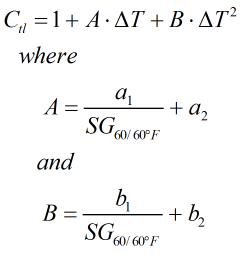# C200 – ASTM-IP Table 24:1952 – Volume Reduction to 60°F

## Description

This calculates the specific gravity at an alternate temperature from specific gravity at 60°F and the alternate temperature. Note this calculation is only valid for portion of the table where specific gravity input is 0.600 and above.

Report on the Development, Construction, Calculation and Preparation of the ASTM-IP Petroleum Measurement Tables – ASTM D 1250; IP 200 (1960)

ASTM-IP Petroleum Measurement Tables – ASTM D 1250; IP 200 (1952)

Kelton calculation reference C200

FLOCALC calculation reference F086

## Options

### Precision

• Full
• Rounding

If rounding is selected the output is rounded to 4 decimal places as per Table 24 otherwise values are displayed to full precision.

## Calculation

### Temperature Correction

The temperature correction is calculated by :Where ΔT = Difference in temperature compared to base temperature in °F SG60/60°F = Specific gravity at 60°F a1 = Constant from Table III a2 = Constant from Table III b1 = Constant from Table III b2 = Constant from Table III